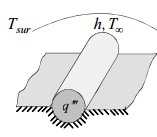### Analyze the temperature distribution in the cable

Assignment Help Electrical Engineering
##### Reference no: EM131284386

A long electric cable generates heat at a rate qc. Half the cable is buried underground while the other half exchanges heat by radiation and convection. The ambient and surroundings temperatures are and respectively. The heat transfer coefficient is h.

Neglecting the thickness of the electrical insulation layer and heat loss to the ground, select a model to analyze the temperature distribution in the cable and write the heat equation and boundary conditions.#### Write two dimensional steady state heat equation

A rectangular plate of length L and height H slides down an inclined surface with a velocity U.- Write the two-dimensional steady state heat equation and boundary conditions.

#### Write the two dimensional steady state heat equation

The outside temperature and heat transfer coefficient are and The two plane surfaces are maintained at uniform temperature. Write the two-dimensional steady state heat equat

#### Write the steady state two dimensional heat equation

The heat transfer coefficient is h, emissivity H, ambient temperature T and surroundings temperature is T Use a simplified radiation model and assume that the cable is infi

#### Determine steady state temperature distribution in two plate

The surface of the stationary plate is insulated while that of the moving plate is maintained at constant temperature T. Determine the steady state temperature distribution

#### Design a zener regulator to meet these specifications

A TIL312 is a seven-segment indicator. Each segment has a voltage drop between 1.5 and 2 V at 20 mA. The supply voltage is +5 V. Design a seven-segment display circuit contr

#### What is the collector-emitter voltage in this circuit

When the base and collector supplies are equal, the transistor can be drawn as shown in Fig. 6-34b. What is the collector-emitter voltage in this circuit? The transistor pow

#### Draw another base-biased circuit

Draw another base-biased circuit. Draw a load line for the circuit and tell me how to calculate the saturation and cutoff points. Discuss the effects of a changing current g

#### Calculate the transistor currents and voltages

Draw a transistor circuit with a CE connection. Now, what kind of troubles can you get with a circuit like this and what measurements would you make to isolate each trouble?

### Write a Review